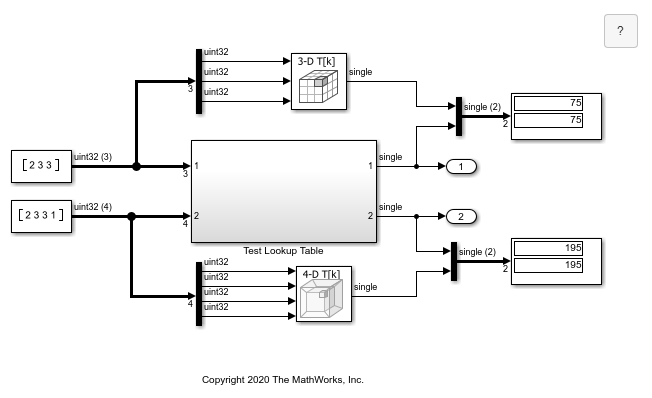# Call Legacy Lookup Table Functions Using C Function Block

This example shows how to use the C Function block to call legacy C functions that implement n-dimensional table lookups.

In this example the legacy C functions are defined in lookupTable.h, and implemented in directLookupTableND.c. The type definitions used in this example can be found in your_types.h.

In the model, the header file and the source file are specified in Model Configuration Parameters > Simulation Target. Now the C function can be called in the C Function block. The prototype of the function being called in this example is:

`FLT directLookupTableND(const FLT *tableND, const UINT32 nbDims, const UINT32 *tableDims, const UINT32 *tableIdx)`

where `FLT` is a type definition to a floating-point type, and `UINT32` is a type definition to an unsigned 32-bit integer.

This example defines two macro functions that use the function `directLookupTableND`, which are called using the C Function blocks. They are:

• `DirectLookupTable3D` - 3D lookup table

• `DirectLookupTable4D` - 4D lookup table

Both these functions take the following input arguments:

• `const FLT *tableND` - Table

• `const UINT32 *tableDims` - Size of the table

• `const UINT32 *tableIdx` - Table index

where the `tableND` and `tableDims` are mapped to C Function block parameters and `tableIdx` is the input to the block. The value returned by the legacy C function is the output of the block.

```model = 'slexCFunctionLookupTable'; open_system(model); evalc('sim(model)'); slcc('clearCustomCodeModules'); ```